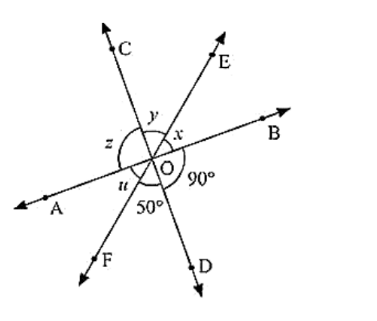# In the below fig. three coplanar lines intersect at a point O, forming angles as shown in the figure.`
Question:

In the below fig. three coplanar lines intersect at a point O, forming angles as shown in the figure. Find the values of x, y, z and u.Solution:

Vertically opposite angles are equal

So ∠SOD = z = 90°

∠DOF = y = 50°

Now, x + y + z = 180 [Linear pair]

x + y + z = 180

90 + 50 + x = 180

x = 180 - 140

x = 40

Hence values of x, y, z and u are 40, 50, 90 and 40 respectively in degrees.